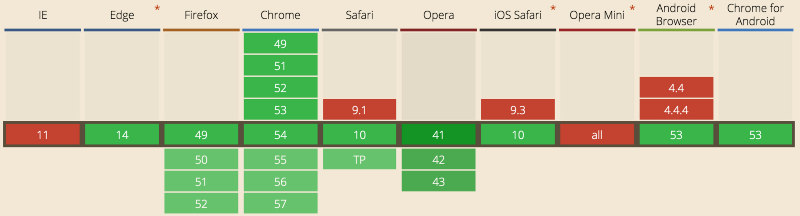# 使用虚拟dom和JavaScript构建完全响应式的UI框架

• 踏歌
• 8742 访问

#### UI框架``````**import** { create, h } **from 'virtual-dom'**;

**const** _render_ = (state) => {
**const** children = state.**list**.map(t => h(**'li'**,{},[t]));
**return** h(**'ul'**, {}, children);
};

**const** INITIAL_STATE = {
**list**:[**'first'**,**'second'**]
};

**let** tree = _render_(INITIAL_STATE);
**let** rootNode = create(tree);

document.**body**.appendChild(rootNode);
``````

``````**const** _updateDom_ = (state) => {
**const** newTree = _render_(state);
**const** patches = diff(tree, newTree);

tree = newTree;
rootNode = patch(rootNode, patches);
};
``````

#### 响应式状态管理库``````state.list = […state.list,’Another Element’];
``````

Proxy 对象用来为基础操作（例如：属性查找、赋值、枚举、方法调用等）定义用户自定义行为。Oh you IE…

``````**export default** (target) => {
**const** loggingHandler = {
get: **function** (target,name) {
**const** value = target[name];
**console**.log(**`getting** \${name}**:** \${value}**`**);
**return** value;
},
set: **function** (target,name,value) {
**console**.log(**`setting** \${name}**:** \${value}**`**);
target[name] = value;
**return true**;
}
};

**return new** Proxy(target,loggingHandler);
};
``````

``````**const** INITIAL_STATE = {
**startValue**:0
};

**const** state = _loggable_(INITIAL_STATE);

**const** value = state.**startValue**; //prints 'getting startValue: 0'

state.**startValue** = 1; //prints 'setting startValue: 1'
``````

``````**export default** ({target,listener}) => {
**let** observable;

**const** _set_ = (target,name,value) => {
target[name] = value;
listener(observable);
**return true**;
};

**const** _get_ = (target,name) => {
**return** Object.freeze(target[name]);
};

**const** handler = {
_set_,
_get_ };

observable = **new** Proxy(target,handler);

**return** observable;
};
``````

``````**import** { patch, create, diff } **from 'virtual-dom'**;
**import** { _render_ } **from './view'**;
**import** _loggable_ **from './loggable'**;
**import** _observable_ **from './observable'**;

**const** _updateDom_ = (state) => {
**const** newTree = _render_(state);
**const** patches = diff(tree, newTree);

tree = newTree;
rootNode = patch(rootNode, patches);
};

**const** INITIAL_STATE = {
_};

**const** state = _observable_({
**target**:_loggable_(INITIAL_STATE),
listener:_updateDom
_});

**let** tree = _render_(state);
**let** rootNode = create(tree);

document.**body**.appendChild(rootNode);
``````

#### 后记